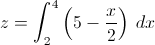# AP Calculus AB & BC: Homework Help Resource Final Exam

Free Practice Test Instructions:

Choose your answer to the question and click 'Continue' to see how you did. Then click 'Next Question' to answer the next question. When you have completed the free practice test, click 'View Results' to see your results. Good luck!

#### Question 1 1. Evaluate and solve the following integral.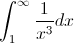#### Question 2 2. Differentiate f(x).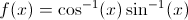#### Question 3 3. What is the one-term left-side Riemann sum for y=f(x) from 0 to 2 for the graph below?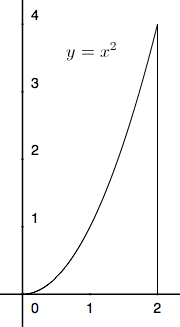#### Question 4 4. Given a velocity v(t) as defined below and a starting position of x=1 at t=0, what is the equation for your position as a function of time?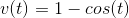#### Question 5 5. Evaluate the following integral.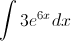#### Question 6 6. Using the given data, solve for z.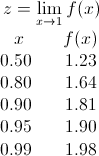#### Question 8 8. Which of the following is equivalent to the expression below?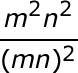#### Question 9 9. Find the derivative of p(t).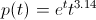#### Question 10 10. Use the following information to answer the question.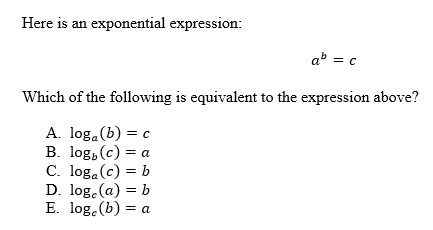#### Question 11 11. Solve the exponential equation below for x: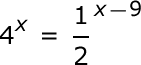#### Question 13 13. Use the graph and integral to find z.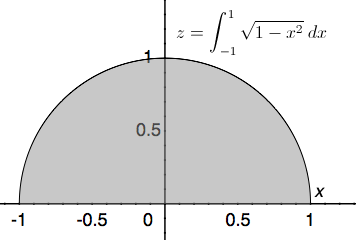#### Question 14 14. Graph the following to find z.